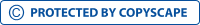Costs, Average variable cost, Total costView larger

# Costs, Average variable cost, Total cost

547478

• College
• 725

Short excerpt:

For the Total Variable Cost, we multiply the number of workers by the average wage per worker. From this result, we add Other Variable Costs to find the Total Variable Cost. The number of workers and their wages are classed as variables because can fluctuate. Other Variable Costs typically remain the same. The formula is shown below:For the Average Variable Cost, we take the above sum (Total Variable Cost) and divide it by the units of output per day. This is an extension of the first equation because it takes the Total Variable Cost and breaks it down into Variable Cost per unit of output. This is an important figure to know because from this, the revenue price for each product can be calculated. The formula is shown below:To determine Average Total Cost, we need to add the Total FixedBy buying this product you can collect up to 28 loyalty points. Your cart will total 28 loyalty points that can be converted into a voucher of \$0.28.

\$2.90